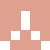## 【六一活动任务】黑公主

6.0 限时 1985℃

•2020-6-1
2
回到灵堂按照坐标都找不到巫婆，怎么搞、
•2020-6-1
3
黑公主
Point1=时光隧道[B]:233:125
Point2=森林:31:192
Point3=小矮人:199:155
Point4=打字[公主为什么这么黑]拿坐标
Point5=[回城]
Point6=进城堡:154:101
Point7=召唤之间:48:85
Point8=回廊:27:9
Point9=回廊二:23:20
Point10=灵堂:10:8
Point11=传送:15:17[[[迷宫找巫婆]]
Point20=打完回城
Point21=时光隧道[B]:233:125
Point22=森林:31:192
Point23=黑公主:202:156
Point24=======<随机迷宫自行修改>=======
Point25=1F:28:28
Point26=2F:13:18
Point27=3F:18:45
Point28=4F:49:30
Point29=5F:34:27
Point30=6F:17:31
Point31=7F:22:28
Point32=boss:23:39